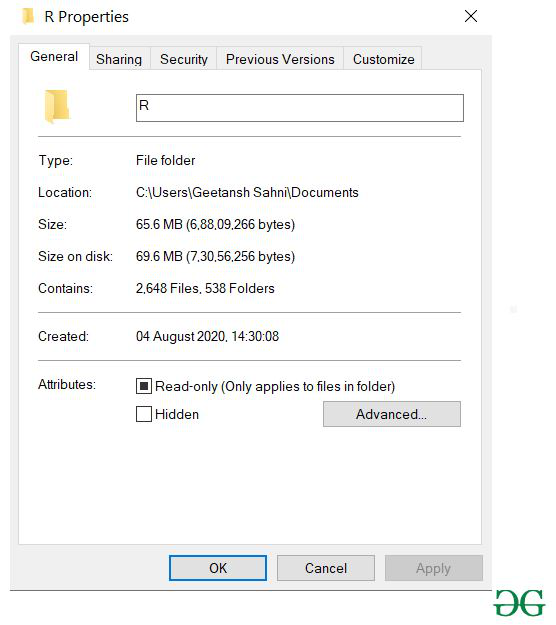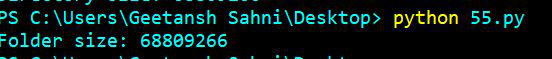# How to get size of folder using Python?

In this article, we are going to discuss various approaches to get the size of a folder using python.

To get the size of a directory, the user has to walk through the whole folder and add the size of each file present in that folder and will show the total size of the folder.

Steps to be followed:

• Import required module.
• Create a variable size and assign 0 to it.
• Assign path of the folder.
• Scan the folder and get the size of each file in the folder and add it to size.
• Display the total size of the folder.

We are going to use the below folder to depict each approach:Method #1: Using os.walk() + os.path.getsize()

In this approach, we will iterate each file present in the folder using os.walk() and then compute and add the size of each scanned file using os.path.getsize().

## Python3

 `# import module` `import` `os`   `# assign size` `size ``=` `0`   `# assign folder path` `Folderpath ``=` `'C:/Users/Geetansh Sahni/Documents/R'`   `# get size` `for` `path, dirs, files ``in` `os.walk(Folderpath):` `    ``for` `f ``in` `files:` `        ``fp ``=` `os.path.join(path, f)` `        ``size ``+``=` `os.path.getsize(fp)`   `# display size` `print``(``"Folder size: "` `+` `str``(size))`

Output:Method #2: Using os.walk() + os.stat()

In this approach, we will iterate each file present in the folder using os.walk(). The os.stat() method returns file properties, now using os.stat().st_size we can compute the file size. Hence, the total folder size can be calculated.

## Python3

 `# import module` `import` `os`   `# assign size` `size ``=` `0`   `# assign folder path` `Folderpath ``=` `'C:/Users/Geetansh Sahni/Documents/R'`     `# get size` `for` `path, dirs, files ``in` `os.walk(Folderpath):` `    ``for` `f ``in` `files:` `        ``fp ``=` `os.path.join(path, f)` `        ``size ``+``=` `os.stat(fp).st_size`   `# display size        ` `print``(``"Folder size: "` `+` `str``(size))`

Output:Method #3: Using os.scandir() + os.path.getsize()

Here, we will scan each file present in the folder using os.scandir() and then we will compute each file size using os.path.getsize(), then we will store the total added size in a variable.

## Python3

 `# import module` `import` `os`   `# assign size` `size ``=` `0`   `# assign folder path` `Folderpath ``=` `'C:/Users/Geetansh Sahni/Documents/R'`      `# get size` `for` `ele ``in` `os.scandir(Folderpath):` `    ``size``+``=``os.path.getsize(ele)` `    `  `print``(size)`

Output:Method #4: Using os.scandir() + os.stat()

Here, we will scan each file present in the folder using os.scandir(). The os.stat() method returns file properties, now using os.stat().st_size we can compute the file size. Hence, the total folder size can be calculated.

## Python3

 `# import module` `import` `os`   `# assign size` `size ``=` `0`   `# assign folder path` `Folderpath ``=` `'C:/Users/Geetansh Sahni/Documents/R'`     `# get size` `for` `ele ``in` `os.scandir(Folderpath):` `    ``size``+``=``os.stat(ele).st_size` `    `  `print``(size)`

Output:Whether you're preparing for your first job interview or aiming to upskill in this ever-evolving tech landscape, GeeksforGeeks Courses are your key to success. We provide top-quality content at affordable prices, all geared towards accelerating your growth in a time-bound manner. Join the millions we've already empowered, and we're here to do the same for you. Don't miss out - check it out now!